<< Previous    1...   2  3    5  6  ...17    Next >>

Chokmah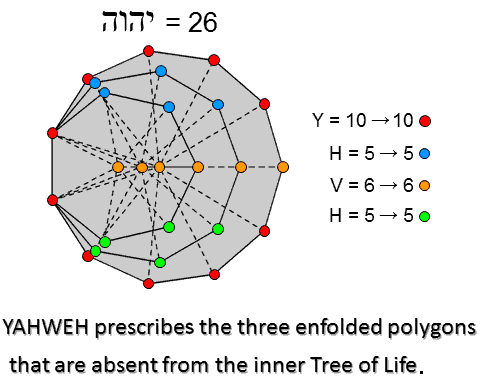Including the two endpoints of the root edge and the centres of the 3 polygons, the 27 triangular sectors have 26 corners. This is the number value of YAHWEH, the Godname of Chokmah. The letter values of YHVH are the numbers of yods belonging to 4 sets. The value 6 of the letter vav (V) denotes the 6 orange yods on the central horizontal axis; the letter value 5 of the two letters he denotes the 5 blue or green corners of the heptagon and nonagon; the letter value 10 of the letter yod denotes the 10 red corners of the undecagon on either side of the horizontal axis.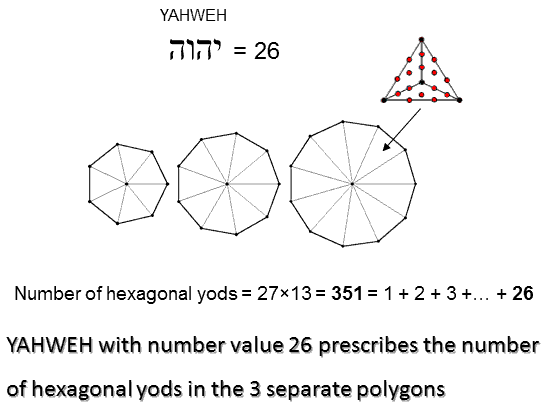The 3 separate Type B polygons have 351 hexagonal yods. This is the 26th triangular number: 351 = 1 + 2 + 3 + ... + 26. YAHWEH with number value 26 prescribes the 3 separate polygons when they are Type B.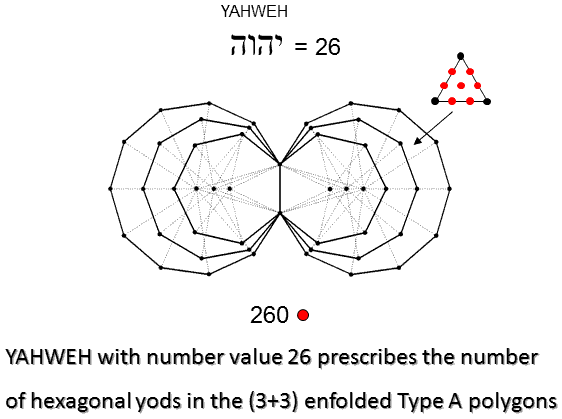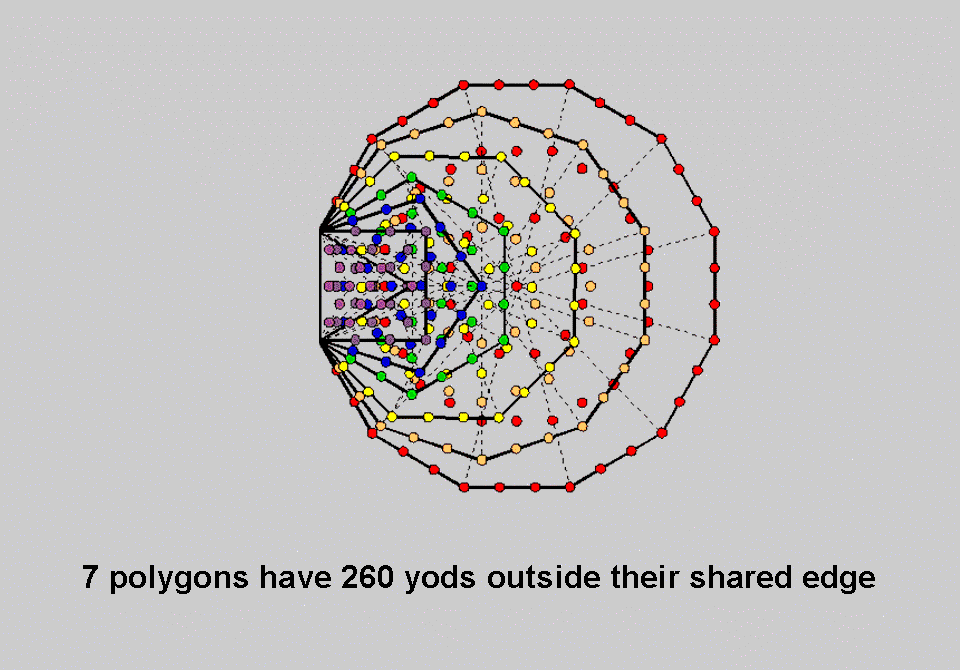The 3 enfolded Type A polygons have 131 hexagonal yods, where 131 is the number value of Samael, the Archangel of Geburah. The (3+3) enfolded Type A polygons have 260 (=26×10) hexagonal yods. YAHWEH prescribes the hexagonal yod population of the (3+3) enfolded polygons. There are 260 yods outside the root edge of the 7 enfolded Type A polygons making up the inner Tree of Life. 130 hexagonal yods are associated with each set of 3 enfolded polygons. Their counterpart in the 7 enfolded polygons are the 130 yods outside the root edge in the triangle, square, pentagon & dodecagon and the 130 yods outside the root edge in the hexagon, octagon & decagon, provided that the centre of the hexagon is counted as belonging to this polygon rather than to the triangle, one of whose corners coincides with it, and when the centre of the decagon is regarded as belonging to this polygon rather than to the pentagon, one of whose corners coincides with it (for proof, see Table 5 in Article 64).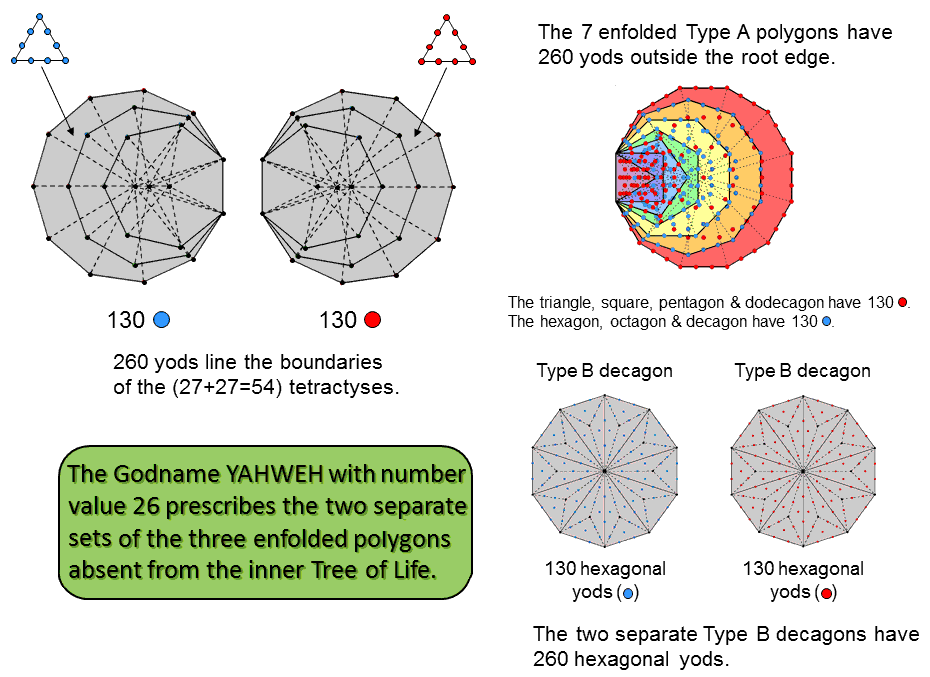The 3 enfolded Type A polygons have 27 sectors with 52 sides and 26 corners. The number of yods lining the sides of the 27 tetractyses = 26 + 52×2 = 130. The two separate sets of 3 enfolded polygons have (130+130=260 = 26×10) yods lining the (52+52=104) sides of the (27+27=54) tetractyses. YAHWEH prescribes the boundaries of the tetractys sectors of the 3 enfolded polygons. This 130:130 division corresponds to the 130 red yods in the triangle, square, pentagon & dodecagon and the 130 blue yods in the hexagon, octagon & decagon. The 130:130 division appears in the 130 hexagonal yods in each of the two separate Type B decagons that belong to the inner Tree of Life. (130−4=126) yods line the 27 sectors of the 3 enfolded Type A polygons outside the root edge. The number of yods lining the (27+27=54) sectors of the (3+3) enfolded polygons = 4 + 2×126 = 256 = 44. This beautiful property displays "design" in that it is par excellence expressed by the Tetrad, as are all parameters of holistic systems.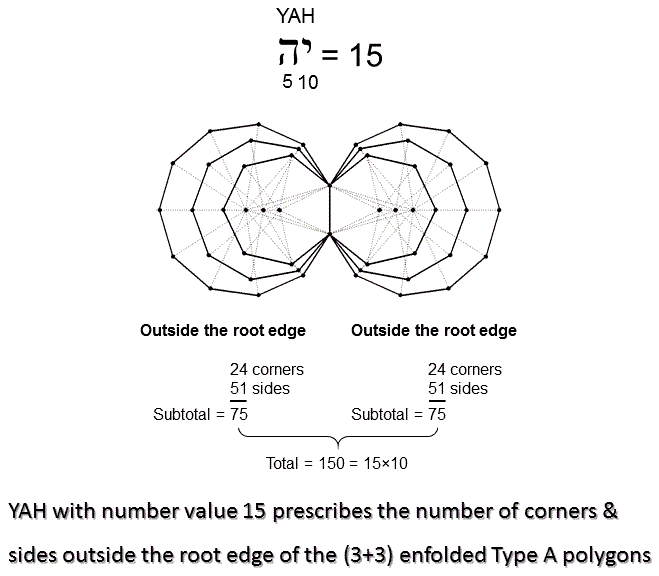According to Table 2, the number of corners & sides in the 3 enfolded Type A polygons = 78. Outside their root edge are 75 corners & sides. Outside the root edge of the (3+3) enfolded Type A polygons are (75×2=150=15×10) corners & sides. YAH, the older version of YAHWEH with number value 15, prescribes the (3+3) enfolded Type A polygons. YAH also prescribes the 3 enfolded polygons because, as calculated here, 152 yods surrounding their centres (including two yods in the shared root edge) can be associated with each set of 3 enfolded Type A polygons. This means that 150 (=15×10) yods outside the root edge surround their centres, YAH prescribes both the number of corners & sides of the sectors of the (3+3) enfolded polygons and the yod population of each set outside their root edge.

 << Previous    1...   2  3    5  6  ...17    Next >>

Home